# 万字总结 JS数据结构与常用的算法# 一、前言

• 第一，是我在今年的flag里明确说到我会学这个东西
• 第二，学了这些，对自己以后在工作或者面试也会带来许多好处

# 二、基本概念

## 1. 时间复杂度

• O(1)
``````    let i = 0
i += 1

• O(n)： 如果是 O(1) + O(n) 则还是 O(n)
``````    for (let i = 0; i < n; i += 1) {
console.log(i)
}

• O(n^2)： O(n) * O(n), 也就是双层循环，自此类推： O(n^3)...
``````    for (let i = 0; i < n; i += 1) {
for (let j = 0; j < n; j += 1) {
console.log(i, j)
}
}

• O(logn)： 就是求 log 以 2 为底的多少次方等于 n
``````    // 这个例子就是求2的多少次方会大于i，然后就会结束循环。 这就是一个典型的 O(logn)
let i = 1
while (i < n) {
console.log(i)
i *= 2
}

## 2. 空间复杂度

• O(1)： 单个变量，所以占用永远是 O(1)
``````    let i = 0
i += 1

• O(n)： 声明一个数组， 添加 n 个值， 相当于占用了 n 个空间单元
``````    const arr = []
for (let i = 0; i < n; i += 1) {
arr.push(i)
}

• O(n^2)： 类似一个矩阵的概念，就是二维数组的意思
``````    const arr = []
for (let i = 0; i < n; i += 1) {
arr.push([])
for (let j = 0; j < n; j += 1) {
arr[i].push(j)
}
}

# 三、数据结构

## 1. 栈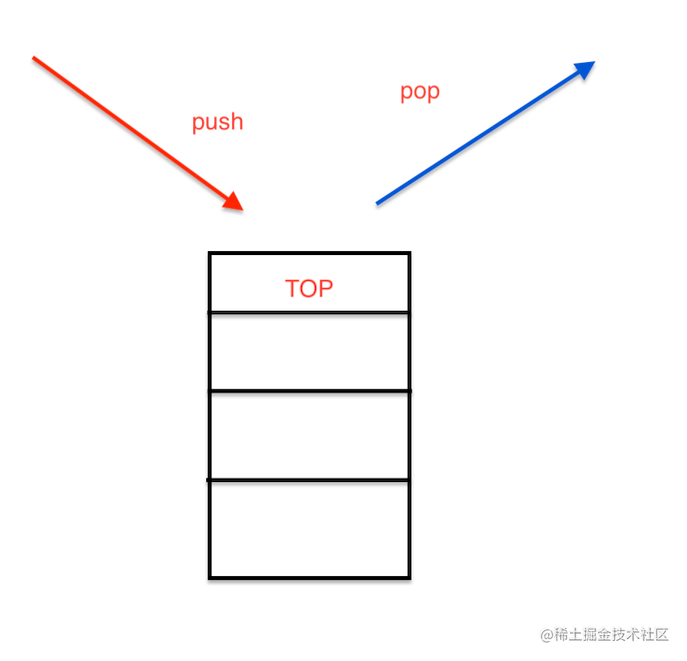js中没有栈的数据类型，但我们可以通过Array来模拟一个

``````const stack = [];

stack.push(1); // 入栈
stack.push(2); // 入栈

const item1 = stack.pop();  //出栈的元素

### 1）十进制转二进制

``````// 时间复杂度 O(n) n为二进制的长度
// 空间复杂度 O(n) n为二进制的长度
const dec2bin = (dec) => {
// 创建一个字符串
let res = "";

// 创建一个栈
let stack = []

// 遍历数字 如果大于0 就可以继续转换2进制
while (dec > 0) {
// 将数字的余数入栈
stack.push(dec % 2);

// 除以2
dec = dec >> 1;
}

// 取出栈中的数字
while (stack.length) {
res += stack.pop();
}

// 返回这个字符串
return res;
};

### 2）判断字符串的有效括号

``````// 时间复杂度O(n) n为s的length
// 空间复杂度O(n)
const isValid = (s) => {

// 如果长度不等于2的倍数肯定不是一个有效的括号
if (s.length % 2 === 1) return false;

// 创建一个栈
let stack = [];

// 遍历字符串
for (let i = 0; i < s.length; i++) {

const c = s[i];

// 如果是左括号就入栈
if (c === '(' || c === "{" || c === "[") {
stack.push(c);
} else {

// 如果不是左括号 且栈为空 肯定不是一个有效的括号 返回false
if (!stack.length) return false

// 拿到最后一个左括号
const top = stack[stack.length - 1];

// 如果是右括号和左括号能匹配就出栈
if ((top === "(" && c === ")") || (top === "{" && c === "}") || (top === "[" && c === "]")) {
stack.pop();
} else {

// 否则就不是一个有效的括号
return false
}
}

}
return stack.length === 0;
};

## 2. 队列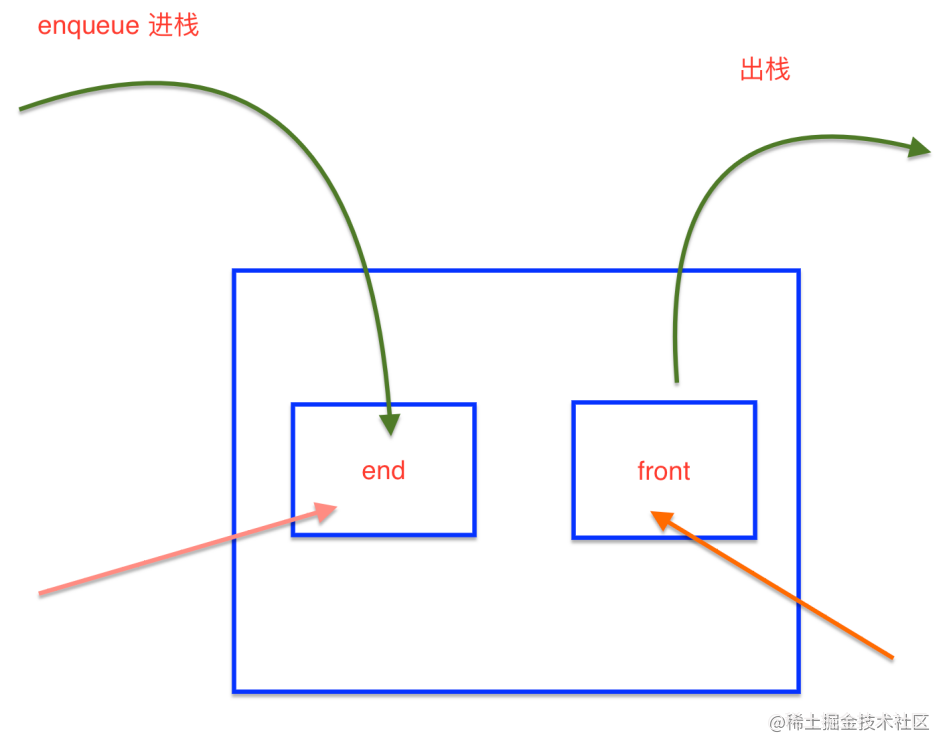``````const queue = [];

// 入队
queue.push(1);
queue.push(2);

// 出队
const first = queue.shift();
const end = queue.shift();

### 1）最近的请求次数

``````var RecentCounter = function () {
// 初始化队列
this.q = [];
};

// 输入 inputs = [[],,,,] 请求间隔为 3000ms
// 输出 outputs = [null,1,2,3,3]

// 时间复杂度 O(n) n为剔出老请求的长度
// 空间复杂度 O(n) n为最近请求的次数
RecentCounter.prototype.ping = function (t) {
// 如果传入的时间小于等于最近请求的时间，则直接返回0
if (!t) return null

// 将传入的时间放入队列
this.q.push(t);

// 如果队头小于 t - 3000 则剔除队头
while (this.q < t - 3000) {
this.q.shift();
}

// 返回最近请求的次数
return this.q.length;
};

## 3. 链表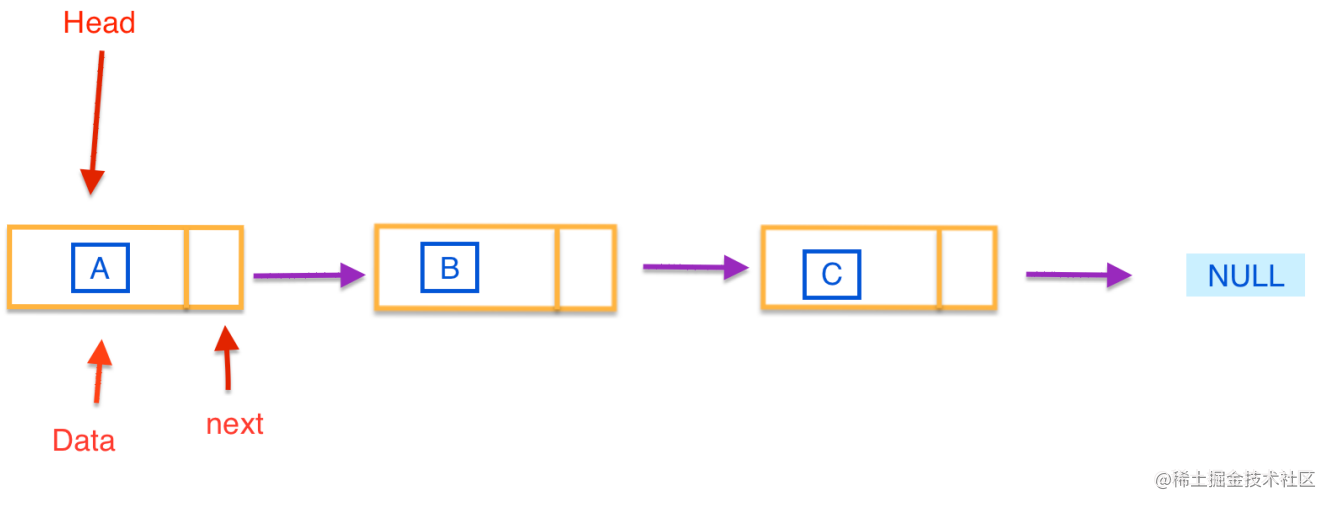js中类似于链表的典型就是原型链, 但是js中没有链表这种数据结构，我们可以通过一个object来模拟链表

``````const a = {
val: "a"
}

const b = {
val: "b"
}

const c = {
val: "c"
}

const d = {
val: "d"
}

a.next = b;
b.next = c;
c.next = d;

//    val: "a",
//    next: {
//        val: "b",
//        next: {
//            val: "c",
//            next: {
//                val: "d",
//                next: null
//            }
//        }
//    }
// }

// 遍历链表
let p = a;
while (p) {
console.log(p.val);
p = p.next;
}

// 插入
const e = { val: 'e' };
c.next = e;
e.next = d;

// 删除
c.next = d;

### 1）手写instanceOf

``````const myInstanceOf = (A, B) => {
// 声明一个指针
let p = A;

// 遍历这个链表
while (p) {
if (p === B.prototype) return true;
p = p.__proto__;
}

return false
}

myInstanceOf([], Object)

### 2）删除链表中的节点

``````// 时间复杂和空间复杂度都是 O(1)
const deleteNode = (node) => {
// 把当前链表的指针指向下下个链表的值就可以了
node.val = node.next.val;
node.next = node.next.next
}

### 3）删除排序链表中的重复元素

``````// 1 -> 1 -> 2 -> 3 -> 3
// 1 -> 2 -> 3 -> null

// 时间复杂度 O(n) n为链表的长度
// 空间复杂度 O(1)
const deleteDuplicates = (head) => {

// 创建一个指针

// 遍历链表
while (p && p.next) {

// 如果当前节点的值等于下一个节点的值
if (p.val === p.next.val) {

// 删除下一个节点
p.next = p.next.next
} else {

// 否则继续遍历
p = p.next
}
}

//  最后返回原来链表
}

### 4）反转链表

``````// 1 -> 2 -> 3 -> 4 -> 5 -> null
// 5 -> 4 -> 3 -> 2 -> 1 -> null

// 时间复杂度 O(n) n为链表的长度
// 空间复杂度 O(1)
var reverseList = function (head) {

// 创建一个指针

// 创建一个新指针
let p2 = null;

// 遍历链表
while (p1) {

// 创建一个临时变量
const tmp = p1.next;

// 将当前节点的下一个节点指向新链表
p1.next = p2;

// 将新链表指向当前节点
p2 = p1;

// 将当前节点指向临时变量
p1 = tmp;
}

// 最后返回新的这个链表
return p2;
}

reverseList(list

## 4. 集合

ES6中有集合 Set类型

``````const arr = [1, 1, 1, 2, 2, 3];

// 去重
const arr2 = [...new Set(arr)];

// 判断元素是否在集合中
const set = new Set(arr);
set.has(2) // true

//  交集
const set2 = new Set([1, 2]);
const set3 = new Set([...set].filter(item => set.has(item)));

### 2）两个数组的交集

``````// 时间复杂度 O(n^2) n为数组长度
// 空间复杂度 O(n)  n为去重后的数组长度
const intersection = (nums1, nums2) => {

// 通过数组的filter选出交集
// 然后通过 Set集合 去重 并生成数组
return [...new Set(nums1.filter(item => nums2.includes(item)))];
}

## 5. 字典

js中有字典数据结构 就是 Map 类型

### 1）两数之和

``````// nums = [2, 7, 11, 15] target = 9

// 时间复杂度O(n) n为nums的length
// 空间复杂度O(n)
var twoSum = function (nums, target) {

// 建立一个字典数据结构来保存需要的值
const map = new Map();
for (let i = 0; i < nums.length; i++) {

// 获取当前的值，和需要的值
const n = nums[i];
const n2 = target - n;

// 如字典中有需要的值，就匹配成功
if (map.has(n2)) {
return [map.get(n2), i];
} else {

// 如没有，则把需要的值添加到字典中
map.set(n, i);
}
}
};

### 2）两个数组的交集

``````// nums1 = [1,2,2,1], nums2 = [2,2]
// 输出：

// 时间复杂度 O(m + n) m为nums1长度 n为nums2长度
// 空间复杂度 O(m) m为交集的数组长度
const intersection = (nums1, nums2) => {
// 创建一个字典
const map = new Map();

// 将数组1中的数字放入字典
nums1.forEach(n => map.set(n, true));

// 创建一个新数组
const res = [];

// 将数组2遍历 并判断是否在字典中
nums2.forEach(n => {
if (map.has(n)) {
res.push(n);

// 如果在字典中，则删除该数字
map.delete(n);
}
})

return res;
};

### 3）字符的有效的括号

``````// 用字典优化

// 时间复杂度 O(n) n为s的字符长度
// 空间复杂度 O(n)
const isValid = (s) => {

// 如果长度不等于2的倍数肯定不是一个有效的括号
if (s.length % 2 !== 0) return false

// 创建一个字典
const map = new Map();
map.set('(', ')');
map.set('{', '}');
map.set('[', ']');

// 创建一个栈
const stack = [];

// 遍历字符串
for (let i = 0; i < s.length; i++) {

// 取出字符
const c = s[i];

// 如果是左括号就入栈
if (map.has(c)) {
stack.push(c)
} else {

// 取出栈顶
const t = stack[stack.length - 1];

// 如果字典中有这个值 就出栈
if (map.get(t) === c) {
stack.pop();
} else {

// 否则就不是一个有效的括号
return false
}

}

}

return stack.length === 0;
};

### 4）最小覆盖字串

``````// 输入：s = "ADOBECODEBANC", t = "ABC"
// 输出："BANC"

// 时间复杂度 O(m + n) m是t的长度 n是s的长度
// 空间复杂度 O(k) k是字符串中不重复字符的个数
var minWindow = function (s, t) {
// 定义双指针维护一个滑动窗口
let l = 0;
let r = 0;

// 建立一个字典
const need = new Map();

//  遍历t
for (const c of t) {
need.set(c, need.has(c) ? need.get(c) + 1 : 1)
}

let needType = need.size

// 记录最小子串
let res = ""

// 移动右指针
while (r < s.length) {

// 获取当前字符
const c = s[r];

// 如果字典里有这个字符
if (need.has(c)) {

// 减少字典里面的次数
need.set(c, need.get(c) - 1);

// 减少需要的值
if (need.get(c) === 0) needType -= 1;
}

// 如果字典中所有的值都为0了 就说明找到了一个最小子串
while (needType === 0) {

// 取出当前符合要求的子串
const newRes = s.substring(l, r + 1)

// 如果当前子串是小于上次的子串就进行覆盖
if (!res || newRes.length < res.length) res = newRes;

// 获取左指针的字符
const c2 = s[l];

// 如果字典里有这个字符
if (need.has(c2)) {
// 增加字典里面的次数
need.set(c2, need.get(c2) + 1);

// 增加需要的值
if (need.get(c2) === 1) needType += 1;
}
l += 1;
}
r += 1;
}
return res
};

## 6. 树

js中没有树 但是可以用 Object 和 Array 构建树

### 1）普通树

``````// 这就是一个常见的普通树形结构
const tree = {
val: "a",
children: [
{
val: "b",
children: [
{
val: "d",
children: [],
},
{
val: "e",
children: [],
}
],
},
{
val: "c",
children: [
{
val: "f",
children: [],
},
{
val: "g",
children: [],
}
],
}
],
}

#### > 深度优先遍历

• 尽可能深的搜索树的分支,就比如遇到一个节点就会直接去遍历他的子节点不会立刻去遍历他的兄弟节点
• 口诀：
• 访问根节点
• 对根节点的 children 挨个进行深度优先遍历
``````// 深度优先遍历
const dfs = (tree) => {
tree.children.forEach(dfs)
};

#### > 广度优先遍历

• 先访问离根节点最近的节点, 如果有兄弟节点就会先遍历兄弟节点再去遍历自己的子节点
• 口诀
• 新建一个队列 并把根节点入队
• 把队头出队并访问
• 把队头的children挨个入队
• 重复第二 、三步 直到队列为空
``````// 广度优先遍历
const bfs = (tree) => {
const q = [tree];

while (q.length > 0) {
const n = q.shift()
console.log(n.val);
n.children.forEach(c => q.push(c))
}
};

### 2）二叉树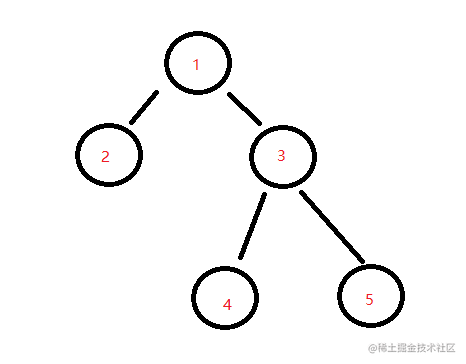`````` const bt = {
val: 1,
left: {
val: 2,
left: null,
right: null
},
right: {
val: 3,
left: {
val: 4,
left: null,
right: null
},
right: {
val: 5,
left: null,
right: null
}
}
}

#### > 二叉树的先序遍历

• 访问根节点
• 对根节点的左子树进行先序遍历
• 对根节点的右子树进行先序遍历
``````// 先序遍历 递归
const preOrder = (tree) => {
if (!tree) return

console.log(tree.val);

preOrder(tree.left);
preOrder(tree.right);
}

// 先序遍历 非递归
const preOrder2 = (tree) => {
if (!tree) return

// 新建一个栈
const stack = [tree];

while (stack.length > 0) {
const n = stack.pop();
console.log(n.val);

// 负负为正
if (n.right) stack.push(n.right);
if (n.left) stack.push(n.left);

}
}

#### > 二叉树的中序遍历

• 对根节点的左子树进行中序遍历
• 访问根节点
• 对根节点的右子树进行中序遍历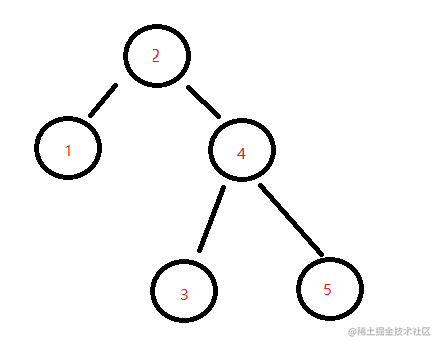``````// 中序遍历 递归
const inOrder = (tree) => {
if (!tree) return;
inOrder(tree.left)
console.log(tree.val);
inOrder(tree.right)
}

// 中序遍历 非递归
const inOrder2 = (tree) => {
if (!tree) return;

// 新建一个栈
const stack = [];

// 先遍历所有的左节点
let p = tree;
while (stack.length || p) {

while (p) {
stack.push(p)
p = p.left
}

const n = stack.pop();
console.log(n.val);

p = n.right;
}
}

#### > 二叉树的后序遍历

• 对根节点的左子树进行后序遍历
• 对根节点的右子树进行后序遍历
• 访问根节点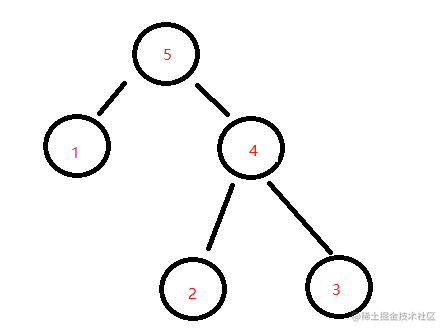``````// 后序遍历 递归
const postOrder = (tree) => {
if (!tree) return

postOrder(tree.left)
postOrder(tree.right)
console.log(tree.val)
};

// 后序遍历 非递归
const postOrder2 = (tree) => {
if (!tree) return

const stack = [tree];
const outputStack = [];

while (stack.length) {
const n = stack.pop();
outputStack.push(n)
// 负负为正
if (n.left) stack.push(n.left);
if (n.right) stack.push(n.right);

}

while (outputStack.length) {
const n = outputStack.pop();
console.log(n.val);
}
};

#### > 二叉树的最大深度

``````// 给一个二叉树，需要你找出其最大的深度，从根节点到叶子节点的距离

// 时间复杂度 O(n) n为树的节点数
// 空间复杂度 有一个递归调用的栈 所以为 O(n) n也是为二叉树的最大深度
var maxDepth = function (root) {
let res = 0;

// 使用深度优先遍历
const dfs = (n, l) => {
if (!n) return;
if (!n.left && !n.right) {
// 没有叶子节点就把深度数量更新
res = Math.max(res, l);
}
dfs(n.left, l + 1)
dfs(n.right, l + 1)
}

dfs(root, 1)

return res
}

#### > 二叉树的最小深度

``````// 给一个二叉树，需要你找出其最小的深度， 从根节点到叶子节点的距离

// 时间复杂度O(n) n是树的节点数量
// 空间复杂度O(n) n是树的节点数量
var minDepth = function (root) {
if (!root) return 0

// 使用广度优先遍历
const q = [[root, 1]];

while (q.length) {
// 取出当前节点
const [n, l] = q.shift();

// 如果是叶子节点直接返回深度就可
if (!n.left && !n.right) return l
if (n.left) q.push([n.left, l + 1]);
if (n.right) q.push([n.right, l + 1]);
}

}

#### > 二叉树的层序遍历``````// 需要返回 [, [2,3], [4,5]]

// 时间复杂度 O(n) n为树的节点数
// 空间复杂度 O(n)
var levelOrder = function (root) {
if (!root) return []

// 广度优先遍历
const q = [root];
const res = [];
while (q.length) {
let len = q.length

res.push([])

// 循环每层的节点数量次
while (len--) {
const n = q.shift();

res[res.length - 1].push(n.val)

if (n.left) q.push(n.left);
if (n.right) q.push(n.right);
}

}

return res
};

## 7. 图

js中可以利用Object和Array构建图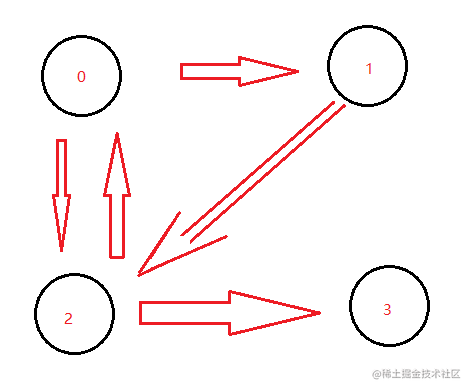``````// 上图可以表示为
const graph = {
0: [1, 2],
1: ,
2: [0, 3],
3: 
}

// 深度优先遍历，对根节点没访问过的相邻节点挨个进行遍历
{
// 记录节点是否访问过
const visited = new Set();
const dfs = (n) => {

// 遍历相邻节点
graph[n].forEach(c => {
// 没访问过才可以，进行递归访问
if(!visited.has(c)){
dfs(c)
}
});
}

// 从2开始进行遍历
dfs(2)
}

// 广度优先遍历
{
const visited = new Set();
// 新建一个队列， 根节点入队， 设2为根节点
const q = ;
while (q.length) {

// 队头出队，并访问
const n = q.shift();
console.log(n);
graph[n].forEach(c => {

// 对没访问过的相邻节点入队
if (!visited.has(c)) {
q.push(c)
}
})
}
}

### 1）有效数字

``````// 生成数字关系图 只有状态为 3 5 6 的时候才为一个数字
const graph = {
0: { 'blank': 0, 'sign': 1, ".": 2, "digit": 6 },
1: { "digit": 6, ".": 2 },
2: { "digit": 3 },
3: { "digit": 3, "e": 4 },
4: { "digit": 5, "sign": 7 },
5: { "digit": 5 },
6: { "digit": 6, ".": 3, "e": 4 },
7: { "digit": 5 },
}

// 时间复杂度 O(n) n是字符串长度
// 空间复杂度 O(1)
var isNumber = function (s) {

// 记录状态
let state = 0;

// 遍历字符串
for (c of s.trim()) {
// 把字符进行转换
if (c >= '0' && c <= '9') {
c = 'digit';
} else if (c === " ") {
c = 'blank';
} else if (c === "+" || c === "-") {
c = "sign";
} else if (c === "E" || c === "e") {
c = "e";
}

// 开始寻找图
state = graph[state][c];

// 如果最后是undefined就是错误
if (state === undefined) return false
}

// 判断最后的结果是不是合法的数字
if (state === 3 || state === 5 || state === 6) return true
return false
};

## 8. 堆

js通常使用数组来表示堆

• 左侧子节点的位置是 2*index + 1
• 右侧子节点的位置是 2*index + 2
• 父节点的位置是 (index - 1) / 2 , 取余数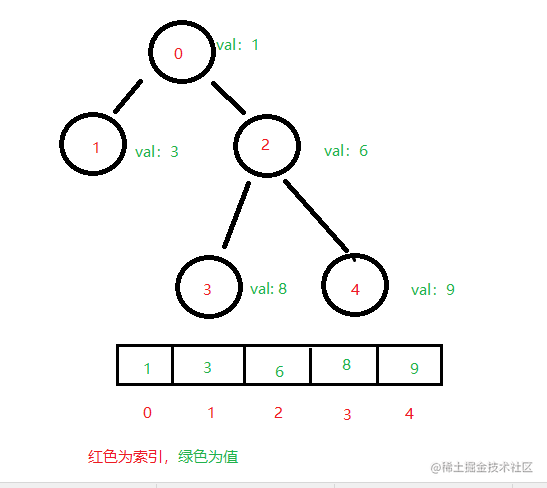### 2）JS实现一个最小堆

``````// js实现最小堆类
class MinHeap {
constructor() {
// 元素容器
this.heap = [];
}

// 交换节点的值
swap(i1, i2) {
[this.heap[i1], this.heap[i2]] = [this.heap[i2], this.heap[i1]]
}

//  获取父节点
getParentIndex(index) {
// 除以二， 取余数
return (index - 1) >> 1;
}

// 获取左侧节点索引
getLeftIndex(i) {
return (i << 1) + 1;
}

// 获取右侧节点索引
getRightIndex(i) {
return (i << 1) + 2;
}

// 上移
shiftUp(index) {
if (index == 0) return;

// 获取父节点
const parentIndex = this.getParentIndex(index);

// 如果父节点的值大于当前节点的值 就需要进行交换
if (this.heap[parentIndex] > this.heap[index]) {
this.swap(parentIndex, index);

// 然后继续上移
this.shiftUp(parentIndex);
}
}

// 下移
shiftDown(index) {
// 获取左右节点索引
const leftIndex = this.getLeftIndex(index);
const rightIndex = this.getRightIndex(index);

// 如果左子节点小于当前的值
if (this.heap[leftIndex] < this.heap[index]) {

// 进行节点交换
this.swap(leftIndex, index);

// 继续进行下移
this.shiftDown(leftIndex)
}

// 如果右侧节点小于当前的值
if (this.heap[rightIndex] < this.heap[index]) {
this.swap(rightIndex, index);
this.shiftDown(rightIndex)
}
}

// 插入元素
insert(value) {
// 插入到堆的底部
this.heap.push(value);

// 然后上移： 将这个值和它的父节点进行交换，知道父节点小于等于这个插入的值
this.shiftUp(this.heap.length - 1)
}

// 删除堆项
pop() {

// 把数组最后一位 转移到数组头部
this.heap = this.heap.pop();

// 进行下移操作
this.shiftDown(0);
}

// 获取堆顶元素
peek() {
return this.heap
}

// 获取堆大小
size() {
return this.heap.length
}

}

### 2）数组中的第k个最大元素

``````// 输入 [3,2,1,5,6,4] 和 k = 2
// 输出 5

// 时间复杂度 O(n * logK) K就是堆的大小
// 空间复杂度 O(K) K是参数k
var findKthLargest = function (nums, k) {

// 使用上面js实现的最小堆类，来构建一个最小堆
const h = new MinHeap();

// 遍历数组
nums.forEach(n => {

// 把数组中的值依次插入到堆里
h.insert(n);

if (h.size() > k) {
// 进行优胜劣汰
h.pop();
}
})

return h.peek()
};

### 3）前 K 个高频元素

``````// nums = [1,1,1,2,2,3], k = 2
// 输出: [1,2]

// 时间复杂度 O(n * logK)
// 空间复杂度 O(k)
var topKFrequent = function (nums, k) {

// 统计每个元素出现的频率
const map = new Map();

// 遍历数组 建立映射关系
nums.forEach(n => {
map.set(n, map.has(n) ? map.get(n) + 1 : 1);
})

// 建立最小堆
const h = new MinHeap();

// 遍历映射关系
map.forEach((value, key) => {

// 由于插入的元素结构发生了变化，所以需要对 最小堆的类 进行改造一下,改造的方法我会写到最后
h.insert({ value, key })
if (h.size() > k) {
h.pop()
}
})
return h.heap.map(item => item.key)
};

// 改造上移和下移操作即可
// shiftUp(index) {
//   if (index == 0) return;
//   const parentIndex = this.getParentIndex(index);
//   if (this.heap[parentIndex] && this.heap[parentIndex].value > this.heap[index].value) {
//     this.swap(parentIndex, index);
//     this.shiftUp(parentIndex);
//   }
// }
// shiftDown(index) {
//   const leftIndex = this.getLeftIndex(index);
//   const rightIndex = this.getRightIndex(index);

//   if (this.heap[leftIndex] && this.heap[leftIndex].value < this.heap[index].value) {
//     this.swap(leftIndex, index);
//     this.shiftDown(leftIndex)
//   }

//   if (this.heap[rightIndex] && this.heap[rightIndex].value < this.heap[index].value) {
//     this.swap(rightIndex, index);
//     this.shiftDown(rightIndex)
//   }
// }

# 四、常见算法及算法思想

## 1. 排序

`把某个乱序的数组变成升序序或者降序的数组`， js比较常用sort方法进行排序

### 1）冒泡排序

• 比较所有相邻元素，如果第一个比第二个大就交换他们
• 执行一次后可以保证最后一个数字是最大的
• 重复执行 n-1 次，就可以完成排序
``````// 时间复杂度 O(n ^ 2) n为数组长度
// 空间复杂度 O(1)
Array.prototype.bubbleSort = function () {
for (i = 0; i < this.length - 1; i++) {
for (let j = 0; j < this.length - 1 - i; j++) {
if (this[j] > this[j + 1]) {

// 交换数据
[this[j], this[j + 1]] = [this[j + 1], this[j]];
}
}
}
}

### 2）选择排序

• 找到数组中最小的值,选中它并放到第一位
• 接着找到数组中第二小的值,选中它并放到第二位
• 重复上述步骤执行 n-1 次
``````// 时间复杂度：O(n ^ 2) n为数组长度
// 空间复杂度：O(1)
Array.prototype.selectionSort = function () {
for (let i = 0; i < this.length - 1; i++) {
let indexMin = i;

for (let j = i; j < this.length; j++) {

// 如果当前这个元素 小于最小值的下标 就更新最小值的下标
if (this[j] < this[indexMin]) {
indexMin = j;
}
}

// 避免自己和自己进行交换
if (indexMin !== i) {

// 进行交换数据
[this[i], this[indexMin]] = [this[indexMin], this[i]];
}
}
}

### 3）插入排序

• 从第二个数，开始往前比较
• 它大就往后排
• 以此类推进行到最后一个数
``````// 时间复杂度 O(n ^ 2)
Array.prototype.insertionSort = function () {

// 遍历数组 从第二个开始
for (let i = 1; i < this.length; i++) {

// 获取第二个元素
const temp = this[i];

let j = i;
while (j > 0) {

// 如果当前元素小于前一个元素 就开始往后移动
if (this[j - 1] > temp) {
this[j] = this[j - 1];
} else {

// 否则就跳出循环
break
}

// 递减
j--;
}

// 前一位置赋值为当前元素
this[j] = temp;
}
}

### 4）归并排序

• 分： 把数组劈成两半 在递归的对子数组进行分操作，直到分成一个个单独的数
• 合： 把两个树合并为有序数组，再对有序数组进行合并， 直到全部子数组合并为一个完整的数组
``````// 时间复杂度 O(nlogn) 分需要劈开数组，所以是logn， 合则是n
// 空间复杂度 O(n)
Array.prototype.mergeSort = function () {

const rec = (arr) => {
// 递归终点
if (arr.length === 1) return arr

// 获取中间索引
const mid = arr.length >> 1;

// 通过中间下标,进行分割数组
const left = arr.slice(0, mid);
const right = arr.slice(mid);

// 左边和右边的数组进行递归,会得到有序的左数组,和有序的右数组
const orderLeft = rec(left);
const orderRight = rec(right);

// 存放结果的数组
const res = [];

while (orderLeft.length || orderRight.length) {

// 如左边和右边数组都有值
if (orderLeft.length && orderRight.length) {

// 左边队头的值小于右边队头的值 就左边队头出队,否则就是右边队头出队
res.push(orderLeft < orderRight ? orderLeft.shift() : orderRight.shift())
} else if (orderLeft.length) {

// 把左边的队头放入数组
res.push(orderLeft.shift())
} else if (orderRight.length) {

// 把右边的队头放入数组
res.push(orderRight.shift())
}
}

return res
}

const res = rec(this)

// 把结果放入原数组
res.forEach((n, i) => this[i] = n)
}

#### > 合并两个有序链表

``````// 时间复杂度O(n) n为链表1和链表2的长度之和
// 空间复杂度O(1)
var mergeTwoLists = function (list1, list2) {

// 新建一个新链表 作为返回值
const res = {
val: 0,
next: null
}

// 指向新链表的指针
let p = res;

// 建立两个指针
let p1 = list1;
let p2 = list2;

// 遍历两个链表
while (p1 && p2) {

// 如果链表1 小于 链表2的值 就接入链表1的值
if (p1.val < p2.val) {
p.next = p1;

// 需要往后移动
p1 = p1.next;
} else {

// 否则接入链表2的值
p.next = p2;

// 需要往后移动
p2 = p2.next;
}

// p永远要往后移动一位
p = p.next;
}

// 如果链表1或者链表2还有值,就把后面的值全部接入新链表
if (p1) {
p.next = p1;
}
if (p2) {
p.next = p2;
}

return res.next;
};

### 5）快速排序

• 分区： 从数组中任意选择一个 基准， 所有比基准小的元素放在基准前面比基准大的元素放在基准后面
• 递归： 递归的对基准前后的子数组进行分区
``````// 时间复杂度 O(nlogN)
// 空间复杂度 O(1)
Array.prototype.quickSort = function () {
const rec = (arr) => {

// 如果数组长度小于等于1 就不用排序了
if (arr.length <= 1) { return arr }

// 存放基准前后的数组
const left = [];
const right = [];

// 取基准
const mid = arr;

for (let i = 1; i < arr.length; i++) {

// 如果当前值小于基准就放到基准前数组里面
if (arr[i] < mid) {
left.push(arr[i]);
} else {

// 否则就放到基准后数组里面
right.push(arr[i]);
}
}

// 递归调用两边的子数组
return [...rec(left), mid, ...rec(right)];
};

const res = rec(this);
res.forEach((n, i) => this[i] = n);
}

## 2. 搜索

`找出数组中某个元素的下标`，js中通常使用indexOf方法进行搜索

### 1）顺序搜索

• 就比如indexOf方法， 从头开始搜索数组中的某个元素

### 2）二分搜索

• 从数组中的中间位置开始搜索，如果中间元素正好是目标值，则搜索结束
• 如果目标值大于或者小于中间元素，则在大于或者小于中间元素的那一半数组中搜索
• `数组必须是有序的，如不是则需要先进行排序`
``````// 时间复杂度：O(log n)
// 空间复杂度：O(1)
Array.prototype.binarySearch = function (item) {
// 代表数组的最小索引
let low = 0;

// 和最大索引
let higt = this.length - 1;

while (low <= higt) {

// 获取中间元素索引
const mid = (low + higt) >> 1;

const element = this[mid];

// 如果中间元素小于于要查找的元素 就把最小索引更新为中间索引的下一个
if (element < item) {
low = mid + 1
} else if (element > item) {

// 如果中间元素大于要查找的元素 就把最大索引更新为中间索引的前一个
higt = mid - 1;
} else {
// 如果中间元素等于要查找的元素 就返回索引
return mid;
}
}

return -1
}

#### > 猜数字大小

``````// 时间复杂度 O(logn) 分割成两半的 基本都是logn
// 空间复杂度 O(1)
var guessNumber = function (n) {

// 定义范围最小值和最大值
const low = 1;
const high = n;

while (low <= high) {

// 获取中间值
const mid = (low + high) >>> 1;

// 这个方法是 leetcode 中的方法
// 如果返回值为-1 就是小了
// 如果返回值为1  就是大了
// 如果返回值为0  就是找到了
const res = guess(mid);

// 剩下的操作就和二分搜索一样
if (res === 0) {
return mid
} else if (res === 1) {
low = mid + 1;
} else {
high = mid - 1;
}
}
};

## 3. 分而治之

### 1）归并排序

• 分：把数组从中间一分为二
• 解：递归地对两个子数组进行归并排序
• 合：合并有序子数组

### 2）快速排序

• 分：选基准，按基准把数组分成两个子数组
• 解：递归地对两个子数组进行快速排序
• 合：对两个子数组进行合并

### 3）二分搜索

• 二分搜索也属于分而治之这种思想

#### > 分而治之思想： 猜数字大小

``````// 时间复杂度 O(logn)
// 空间复杂度 O(logn) 递归调用栈 所以是logn
var guessNumber = function (n) {

// 递归函数 接受一个搜索范围
const rec = (low, high) => {

// 递归结束条件
if (low > high) return;

// 获取中间元素
const mid = (low + high) >>> 1;

// 判断是否猜对
const res = guess(mid)

// 猜对
if (res === 0) {
return mid
} else if (res === 1) {
// 猜大了
return rec(mid + 1, high)
} else {
// 猜小了
return rec(low, mid - 1)
}
}

return rec(1, n)
};

#### > 分而治之思想： 翻转二叉树

``````// 时间复杂度 O(n) n为树的节点数量
// 空间复杂度 O(h) h为树的高度
var invertTree = function (root) {
if (!root) return null
return {
val: root.val,
left: invertTree(root.right),
right: invertTree(root.left)
}
};

#### > 分而治之思想： 相同的树

``````// 时间复杂度 o(n) n为树的节点数量
// 空间复杂度 o(h) h为树的节点数
var isSameTree = function (p, q) {
if (!p && !q) return true

if (
p && q
&& p.val === q.val
&& isSameTree(p.left, q.left)
&& isSameTree(p.right, q.right)
) return true

return false
};

#### > 分而治之思想： 对称二叉树

``````// 时间复杂度 O(n)
// 空间复杂度 O(n)
var isSymmetric = function (root) {
if (!root) return true
const isMirror = (l, r) => {
if (!l && !r) return true
if (
l && r
&& l.val === r.val
&& isMirror(l.left, r.right)
&& isMirror(l.right, r.left)
) return true
return false
}

return isMirror(root.left, root.right)
};

## 4. 动态规划

### 1）斐波那契数列

``````// 时间复杂度 O(n)
// 空间复杂度 O(n)
function fib(n) {
let dp = [0, 1, 1];
for (let i = 3; i <= n; i++) {

// 当前值等于前两个值之和
dp[i] = dp[i - 1] + dp[i - 2];
}
return dp[n];
}

### 2）爬楼梯

``````// 正在爬楼梯, 需要n阶才能到达楼顶
// 每次只能爬 1 或者 2 个台阶, 有多少中不同的方法可以到达楼顶

// 时间复杂度 O(n) n是楼梯长度
// 空间复杂度 O(1)
var climbStairs = function (n) {
if (n < 2) return 1

let dp0 = 1;
let dp1 = 1

for (let i = 2; i <= n; i++) {
[dp0, dp1] = [dp1, dp1 + dp0]
}

return dp1
};

## 5. 贪心算法

### 1）分发饼干

``````// 每个孩子都有一个胃口g. 每个孩子只能拥有一个饼干
// 输入: g = [1,2,3], s = [1,1]
// 输出: 1
// 三个孩子胃口值分别是1,2,3  但是只有两个饼干,所以只能让胃口1的孩子满足

// 时间复杂度 O(nlogn)
// 空间复杂度 O(1)
var findContentChildren = function (g, s) {
// 对饼干和孩子胃口进行排序
g.sort((a, b) => a - b)
s.sort((a, b) => a - b)

// 是第几个孩子
let i = 0

s.forEach((n) => {
// 如果饼干能满足第一个孩子
if (n >= g[i]) {
// 就开始满足第二个孩子
i += 1
}
})

return i
}

### 2）买卖股票的最佳时机Ⅱ

``````// 时间复杂度 O(n) n为股票的数量
// 空间复杂度 O(1)
var maxProfit = function (prices) {
// 存放利润
const profit = 0;
for (let i = 1; i < prices.length; i++) {

// 不贪 如有更高的利润就直接卖出
if (prices[i] > prices[i - 1]) {
profit += prices[i] - prices[i - 1]
}
}

return profit
};

## 6. 回溯算法

### 1）全排列

``````// 输入 [1, 2, 3]
// 输出 [[1, 2, 3], [1, 3, 2], [2, 1, 3], [2, 3, 1], [3, 1, 2], [3, 2, 1]]

// 时间复杂度 O(n!) n! = 1 * 2 * 3 * ··· * (n-1) * n;
// 空间复杂度 O(n)
var permute = function (nums) {
// 存放结果
const res = [];

const backTrack = (path) => {
// 递归结束条件
if (path.length === nums.length) {
res.push(path)
return
}

// 遍历传入数组
nums.forEach(n => {
// 如果子数组中有这个元素就是死路， 需要回溯回去走其他路
if (path.includes(n)) return;

// 加入到子数组里
backTrack(path.concat(n))
})
}

backTrack([])

return res;
};

### 2）子集

``````// 输入 [1,2,3]
// 输出 [ , , , [1,2,3], [1,3], [2,3], [1,2], [] ]

// 时间复杂度 O(2 ^ N) 每个元素都有两种可能
// 空间复杂度 O(N)
var subsets = function (nums) {
// 存放结果数组
const res = [];

const backTrack = (path, l, start) => {
// 递归结束条件
if (path.length === l) {
res.push(path)
return
}

// 遍历输入的数组长度 起始位置是start
for (let i = start; i < nums.length; i++) {

// 递归调用 需要保证子集的有序, start为 i+1
backTrack(path.concat(nums[i]), l, i + 1)
}
};

// 遍历输入数组长度
for (let i = 0; i <= nums.length; i++) {

// 传入长度 起始索引
backTrack([], i, 0)
}

return res
};

# 五、结语

• _island
1年前•• Chris威
5年前
• Ali2333
12月前• TianTianUp
2年前
• ELab
1年前• snowLu
4年前
• TianTianUp
2年前